The Catastrophe Machine

## 3. The Cusp Catastrophe

The Fold Catastrophe corresponds to the perturbation of y=x3 by the addition of a linear function: y=x3+ax, so as to create (a<0) or destroy (a>0) a local minimum. Similarly the Cusp Catastrophe corresponds to the perturbation of y=x4 by the addition of a quadratic function: y=x4+ax+bx2.

If a is kept fixed at 0 and b is varied from +1 to -1, the single local minumum bifurcates into two. If also a varies through negative values (left-hand column), the bifurcation is avoided and the original minimum ends up on the left. But then if, keeping b at -1, a is moved towards negative values (to the right), the missed bifurcation will manifest itself in a "catastrophic" jump to the right-hand local minimum.
 a=0.6 a=0 a=-0.6 b=1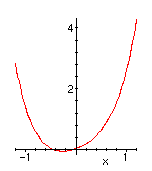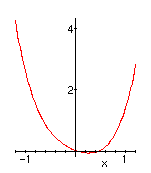b=0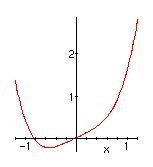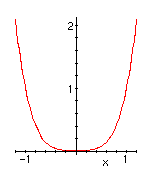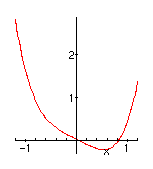b=-1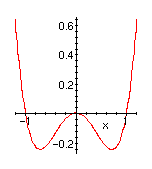As in the fold case, the system will have a discontinuous response at points where a local minimum appears or disappears. A little calculus shows that the system has a single minimum unless -0.5443(-b)3/2 < a < 0.5443(-b)3/2. When the discontinuity locus a=+/- 0.5443(-b)3/2 is graphed in the control space, with coordinates a and b, the plot shows the cusp shape characteristic of this catastrophe. One way to understand the behavior of the system, in analogy with the double well, is to say that the output must track the controls, staying on a surface "above" control space like the one shown at the right. When the right-hand fold is approached from the left, or vice-versa, the output is forced to jump to the other sheet. The membrane in the middle corresponds to the local maximum and is inaccessible.